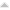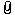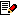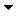How to scale? All  1  2-5From: Michael Gibson 1 Oct 2006  (2 of 5)145.2 In reply to 145.1 Here are some steps that I hope will help explain it. First, let's draw a circle in the top view. Now select the circle, and hit Transform/Scale. You are now running the scale command. In the upper-right corner you'll see a prompt "Pick origin point". This point will define the center around which everything will scale. In this case, let's click on the center of the circle. Now the prompt in the upper-right corner switches to "Pick first reference point". Imagine if there was a grip somewhere that you could grab to scale the circle. This is basically now asking you to place the location of that grip. Let's click anywhere on the circle to place it on the circumference of the circle. Now the prompt is saying "Pick second reference point". This is sort of as if you were dragging the grip that you placed earlier. As you move the mouse around you will see the circle shrink or grow. There are 2 distances involved here - the distance between the origin point and the first reference point, and then the distance between the origin point and the second reference point (these are represented by 2 line segments that you see between the points). The relation between these distances controls the scale factor. If the second distance is twice as big as the first one, then you get a scale factor of 2. If it is half as much, you get a scale factor of 0.5 ... After you pick the origin point, there is also a text entry control up there for "Scale factor". If you type in a number when that is available, it will scale by that amount and you don't have to pick any reference points. For instance, typing in 2.0 there will double the size of the circle. The reason why it asks for these reference points is so you can scale things relative to specific points on existing objects. Here is an example:So in the example, we want to scale the triangle so that it grows by the exact amount so that it touches the corner of the rectangle. We don't really know the exact number of this scale - it looks like somewhere around 2.0, but a little bit less. We don't want to just guess it in this case, we want to be exact. So in step 2, I picked the origin point for the scale at the corner of the triangle. In step 3, I picked the first reference point at the midpoint of the triangle edge. In step4, I picked the second reference point at the corner of the box. MoI has determined the relative difference between these distances and scaled the triangle by that amount. This is the reason why it asks you to place the grip yourself, instead of just giving you an arbitrary one somewhere, with an arbitrary one it might not be in the spot that is of interest to you for a particular operation, such as the midpoint in this particular case. In the future at some point I do want to make some kind of frame with grips already set at the corners all ready for you to grab, instead of making you place the point. That would be easier to understand. But it would also limit the ability to do precise scaling as in the triangle example above. Right now scale is set up to provide this type of exact placement. Please let me know if any of this isn't clear. - Michael Attachments:scale_example.pngReply MoreFrom: el_diablo 2 Oct 2006  (3 of 5)Am I wrong or does 1D Scale seem to scale from origin and not from 1st point?Reply MoreFrom: Michael Gibson 2 Oct 2006  (4 of 5)145.4 In reply to 145.3 Can you post an example of a 1D scale that doesn't seem to work? I just did a quick test and for simple things it seems ok:I think that there are some bugs where it does not work properly when the direction is at certain odd angles though. - Michael Attachments:scale1d_example.pngReply MoreFrom: el_diablo 3 Oct 2006  (5 of 5)The problem being... Attachments:ship_problem.gifReply MoreReply to All
 Show messages: All  1  2-5